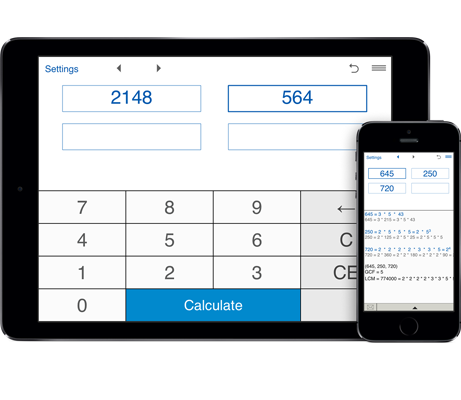# Prime Factor Calculator

Prime factorization of any number (up to 9 digits).
Prime factorization of up to four numbers at once.
Prime factorization in standard and exponential forms.
Finds the GCF and LCM of given numbers.

## Prime Factorization

Prime Factor Calculator is a handy prime factorization calculator that helps you find the prime factors of given numbers.

This prime factor calculator performs the prime factorization of one, two, three and four numbers showing results in the standard form as multiplication of the prime factors and exponential form (power form).

For two, three and four numbers the prime factorization calculator finds the GCF (greatest common factor) and LCM (Least Common Multiple) of numbers.

For prime numbers the prime factor calculator returns the number itself.

#### How to find the prime factors:

Just enter one, two, three or four positive integers and click "Calculate". The prime factorization calculator can handle numbers with up to 9 digits.

#### Examples of prime factorization

Prime factorization of 100:
100 = 2 * 2 * 5 * 5
Prime factorization of 100 using exponents 100 = 22 * 52.

Prime factorization of 76:
76 = 2 * 2 * 19
Prime factorization of 76 using exponents 76 = 22 * 19.

Prime factorization of 48:
48 = 2 * 2 * 2 * 2 * 3
Prime factorization of 48 using exponents 48 = 24 * 3.

Prime factorization of 36:
36 = 2 * 2 * 3 * 3
Prime factorization of 36 using exponents 36 = 22 * 32.

Prime factorization of 18:
18 = 2 * 3 * 3
Prime factorization of 18 using exponents 18 = 2 * 32.

Prime factorization of 20:
20 = 2 * 2 * 5
Prime factorization of 20 using exponents 20 = 22 * 5.

Prime factorization of 24:
24 = 2 * 2 * 2 * 3
Prime factorization of 24 using exponents 24 = 23 * 3.

Prime factorization of 28:
28 = 2 * 2 * 7
Prime factorization of 28 in exponential form 28 = 22 * 7.

Prime factorization of 30:
30 = 2 * 3 * 5

Prime factorization of 32:
32 = 2 * 2 * 2 * 2 * 2
Prime factorization of 32 in exponential form 32 = 25.

Prime factorization of 36:
36 = 2 * 2 * 3 * 3
Prime factorization of 36 in exponential form 36 = 22 * 32.

Prime factorization of 40:
40 = 2 * 2 * 2 * 5
Prime factorization of 40 in exponential form 40 = 23 * 5.

Prime factorization of 42:
42 = 2 * 3 * 7

Prime factorization of 45:
45 = 3 * 3 * 5
Prime factorization of 45 in exponent form 45 = 32 * 5.

Prime factorization of 48:
48 = 2 * 2 * 2 * 2 * 3
Prime factorization of 48 in exponent form 48 = 24 * 3.

Prime factorization of 50:
50 = 2 * 5 * 5
Prime factorization of 50 in exponent form 50 = 2 * 52.

Prime factorization of 52:
52 = 2 * 2 * 13
Prime factorization of 52 in exponent form 52 = 22 * 13.

Prime factorization of 54:
54 = 2 * 3 * 3 * 3
Prime factorization of 54 in exponent form 52 = 2 * 33.

Prime factorization of 56:
56 = 2 * 2 * 2 * 7
Prime factorization of 56 using exponents 56 = 23 * 7.

Prime factorization of 60:
60 = 2 * 2 * 3 * 5
Prime factorization of 60 using exponents 60 = 22 * 3 * 5.

Prime factorization of 63:
63 = 3 * 3 * 7
Prime factorization of 63 in exponent form 63 = 32 * 7.

Prime factorization of 64:
64 = 2 * 2 * 2 * 2 * 2 * 2
Prime factorization of 64 in exponent form 64 = 26.

Prime factorization of 68:
68 = 2 * 2 * 17
Prime factorization of 68 in exponent form 64 = 22 * 17.

Prime factorization of 70:
70 = 2 * 5 * 7

Prime factorization of 75:
75 = 3 * 5 * 5
Prime factorization of 75 in exponent form 75 = 3 * 52.

Prime factorization of 78:
78 = 2 * 3 * 13

Prime factorization of 80:
80 = 2 * 2 * 2 * 2 * 5
Prime factorization of 80 using exponents 80 = 24 * 5.

Prime factorization of 81:
81 = 3 * 3 * 3 * 3
Prime factorization of 81 using exponents 81 = 34.

Prime factorization of 84:
84 = 2 * 2 * 3 * 7
Prime factorization of 84 using exponents 84 = 22 * 3 * 7.

Prime factorization of 85:
85 = 5 * 17

Prime factorization of 90:
90 = 2 * 3 * 3 * 5
Prime factorization of 90 using exponents 90 = 2 * 32 * 5.

Prime factorization of 96:
96 = 2 * 2 * 2 * 2 * 2 * 3
Prime factorization of 96 using exponents 96 = 25 * 3.

Prime factorization of 98:
98 = 2 * 7 * 7
Prime factorization of 96 using exponents 98 = 2 * 72.

Prime factorization of 99:
99 = 3 * 3 * 11
Prime factorization of 99 using exponents 99 = 32 * 11.